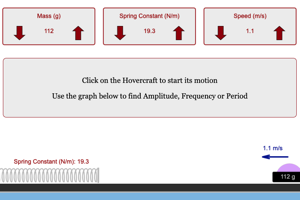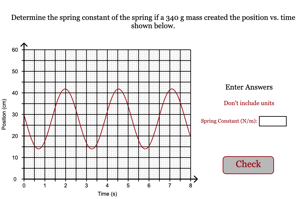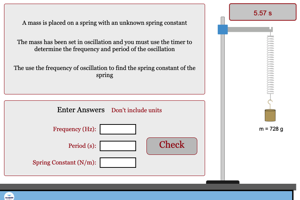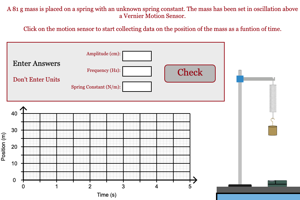Programs for Simple Harmonic Motion
Below are all the programs that might be used with this topic. Click on "See Resources" to see if there are any student directions, lab sheets or other materials that have been created for this program.
Horizontal Oscillations Lab

LabHorizontal Oscillations with Damping Lab

LabOscillations Lab

LabSpring Constant from Oscillation Graph

HomeworkVertical Oscillation with Timer

HomeworkVertical Oscillation Problem

HomeworkEquations of Sine Curves

GameVisualizing Sine Curves

Tool